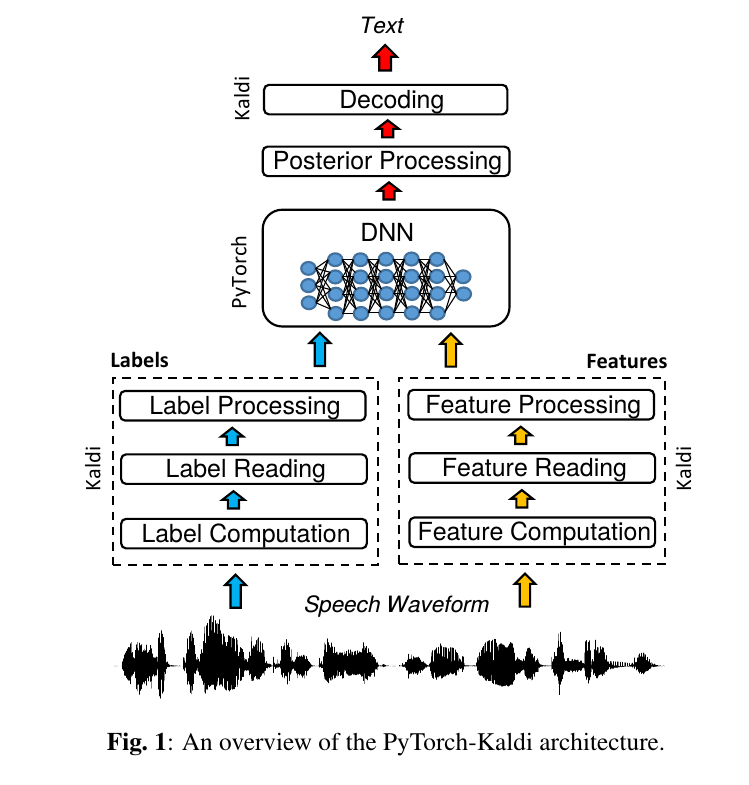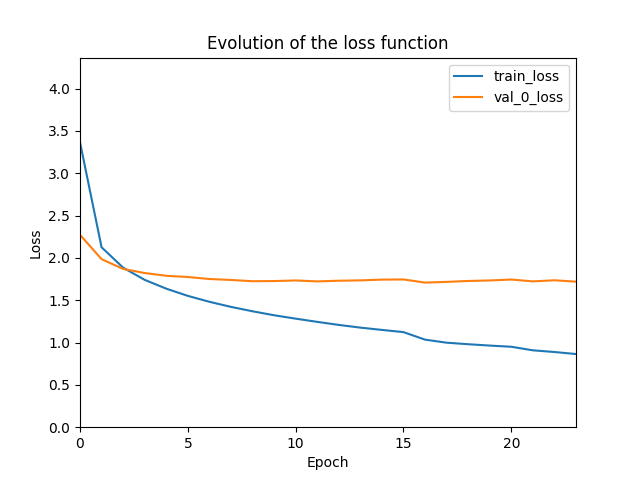# PyTorch-Kaldi简介

Posted by lili on

## 架构图：PyTorch-Kaldi架构

## 简介

PyTorch-Kaldi的目的是作为Kaldi和PyTorch的一个桥梁，它能继承Kaldi的高效和PyTorch的灵活性。PyTorch-Kaldi并不只是这两个工具的粘合剂，而且它还提供了用于构建现代语音识别系统的很多有用特性。比如，代码可以很容易的插入用户自定义的声学模型。此外，用户也可以利用预先实现的网络结果，通过简单的配置文件修改就可以实现不同的模型。PyTorch-Kaldi也支持多个特征(feature)和标签(label)流的融合，使用复杂的网络结构。 它提供完善的文档并且可以在本地或者HPC集群上运行。

• 使用Kaldi的简单接口
• 容易插入(plug-in)自定义模型
• 预置许多常见模型，包括MLP, CNN, RNN, LSTM, GRU, Li-GRU, SincNet
• 基于多种特征、标签和网络结构的复杂模型实现起来非常自然。
• 简单和灵活的配置文件
• 自动从上一次处理的块(chunk)恢复并继续训练
• 自动分块(chunking)和进行输入的上下文扩展
• 多GPU训练
• 可以本地或者在HPC机器上运行
• TIMIT和Librispeech数据集的教程

## 依赖

### Kaldi

export KALDI_ROOT=/home/lili/codes/kaldi
PATH=$KALDI_ROOT/tools/openfst:$PATH
PATH=$KALDI_ROOT/src/featbin:$PATH
PATH=$KALDI_ROOT/src/gmmbin:$PATH
PATH=$KALDI_ROOT/src/bin:$PATH
PATH=$KALDI_ROOT/src/nnetbin:$PATH
export PATH


## 安装

git clone https://github.com/mravanelli/pytorch-kaldi
pip install -r requirements.txt


## TIMIT教程

### 获取数据

NLP很多数据集比如CTB树库等也是LDC提供的，因此也存在同样的问题。不过好在现在流行End-to-End的系统，那些语言学家感兴趣的中间步骤比如词性标注、句法分析其实并没有太多用处。当然这是我的个人看法，Frederick Jelinek曾经说道：”每当我开除一个语言学家，语音识别系统就更准了！” 我觉得也可以这样说：每当系统减掉一个中间环节，NLP系统也更加准确！

### 使用Kaldi进行训练

#### 原理回顾

Kaldi是传统的HMM-GMM，我们希望用神经网络来替代其中的GMM声学模型部分。声学模型可以认为是计算概率$P(X \vert q)$，这里q表示HMM的状态，而X是观察(比如MFCC特征)，但是神经网络是区分性(discriminative)模型，它只能计算$P(q \vert X)$，也就是给定观察，我们可以计算它属于某个状态的概率，也就是进行分类。当然，根据贝叶斯公式：

$P(X | q)=\frac{P(q|X)P(X)}{P(q)} \propto \frac{P(q|X)}{P(q)}$

#### Kaldi训练

lili@lili-Precision-7720:~/codes/kaldi/egs/timit/s5$git diff cmd.sh diff --git a/egs/timit/s5/cmd.sh b/egs/timit/s5/cmd.sh index 6c6dc88..7e3d909 100644 --- a/egs/timit/s5/cmd.sh +++ b/egs/timit/s5/cmd.sh @@ -10,10 +10,10 @@ # conf/queue.conf in http://kaldi-asr.org/doc/queue.html for more information, # or search for the string 'default_config' in utils/queue.pl or utils/slurm.pl. -export train_cmd="queue.pl --mem 4G" -export decode_cmd="queue.pl --mem 4G" +export train_cmd="run.pl --mem 4G" +export decode_cmd="run.pl --mem 4G" # the use of cuda_cmd is deprecated, used only in 'nnet1', -export cuda_cmd="queue.pl --gpu 1" +export cuda_cmd="run.pl --gpu 1"  接着修改修改run.sh里的数据路径timit变量修改成你自己的路径，另外我的机器CPU也不够多，因此把train_nj改小一点。 lili@lili-Precision-7720:~/codes/kaldi/egs/timit/s5$ git diff run.sh
diff --git a/egs/timit/s5/run.sh b/egs/timit/s5/run.sh
index 58bd871..5c322cc 100755
--- a/egs/timit/s5/run.sh
+++ b/egs/timit/s5/run.sh
@@ -28,7 +28,7 @@ numLeavesSGMM=7000
numGaussSGMM=9000

feats_nj=10
-train_nj=30
+train_nj=8
decode_nj=5

echo ============================================================================
@@ -36,8 +36,8 @@ echo "                Data & Lexicon & Language Preparation
echo ============================================================================

#timit=/export/corpora5/LDC/LDC93S1/timit/TIMIT # @JHU
-timit=/mnt/matylda2/data/TIMIT/timit # @BUT
-
+#timit=/mnt/matylda2/data/TIMIT/timit # @BUT
+timit=/home/lili/databak/ldc/LDC/timit/TIMIT
local/timit_data_prep.sh timit || exit 1 local/timit_prepare_dict.sh  最后我们开始训练： cd kaldi/egs/timit/s5 ./run.sh ./local/nnet/run_dnn.sh  #### 强制对齐 我们有两种选择，第一种使用HMM-GMM的对齐来训练PyTorch-Kaldi，对于这种方式，训练数据已经对齐过了(因为训练HMM-DNN就需要对齐)，所以只需要对开发集和测试集再进行对齐： cd kaldi/egs/timit/s5 steps/align_fmllr.sh --nj 4 data/dev data/lang exp/tri3 exp/tri3_ali_dev steps/align_fmllr.sh --nj 4 data/test data/lang exp/tri3 exp/tri3_ali_test  但是更好的是使用HMM-DNN来做对齐，作者使用的是这种方式，这就需要对训练集再做一次对齐了，因为之前的对齐是HMM-GMM做的，不是我们需要的。 steps/nnet/align.sh --nj 4 data-fmllr-tri3/train data/lang exp/dnn4_pretrain-dbn_dnn exp/dnn4_pretrain-dbn_dnn_ali steps/nnet/align.sh --nj 4 data-fmllr-tri3/dev data/lang exp/dnn4_pretrain-dbn_dnn exp/dnn4_pretrain-dbn_dnn_ali_dev steps/nnet/align.sh --nj 4 data-fmllr-tri3/test data/lang exp/dnn4_pretrain-dbn_dnn exp/dnn4_pretrain-dbn_dnn_ali_test  #### 修改PyTorch-Kaldi的配置 我们这里只介绍最简单的全连接网络(基本等价与Kaldi里的DNN)，这个配置文件在PyTorch-Kaldi根目录下，位置是cfg/TIMIT_baselines/TIMIT_MLP_mfcc_basic.cfg。从这个文件名我们可以猜测出这是使用MFCC特征的MLP模型，此外cfg/TIMIT_baselines目录下还有很多其它的模型。这个我们需要修改其中对齐后的目录等数据，请读者参考作者的修改进行修改。 diff --git a/cfg/TIMIT_baselines/TIMIT_MLP_mfcc_basic.cfg b/cfg/TIMIT_baselines/TIMIT_MLP_mfcc_basic.cfg index 6f02075..6e5dc5d 100644 --- a/cfg/TIMIT_baselines/TIMIT_MLP_mfcc_basic.cfg +++ b/cfg/TIMIT_baselines/TIMIT_MLP_mfcc_basic.cfg @@ -15,18 +15,18 @@ n_epochs_tr = 24 [dataset1] data_name = TIMIT_tr fea = fea_name=mfcc - fea_lst=/home/mirco/kaldi-trunk/egs/timit/s5/data/train/feats.scp - fea_opts=apply-cmvn --utt2spk=ark:/home/mirco/kaldi-trunk/egs/timit/s5/data/train/utt2spk ark:/home/mirco/kaldi-trunk/egs/timit/s5/mfcc/cmvn_train.ark ark:- ark:- | add-deltas --delta-order=2 ark:- ark:- | + fea_lst=/home/lili/codes/kaldi/egs/timit/s5/data/train/feats.scp + fea_opts=apply-cmvn --utt2spk=ark:/home/lili/codes/kaldi/egs/timit/s5/data/train/utt2spk ark:/home/lili/codes/kaldi/egs/timit/s5/mfcc/cmvn_train.ark ark:- ark:- | add-deltas --delta-order=2 ark:- ark:- | cw_left=5 cw_right=5 lab = lab_name=lab_cd - lab_folder=/home/mirco/kaldi-trunk/egs/timit/s5/exp/dnn4_pretrain-dbn_dnn_ali + lab_folder=/home/lili/codes/kaldi/egs/timit/s5/exp/dnn4_pretrain-dbn_dnn_ali lab_opts=ali-to-pdf lab_count_file=auto - lab_data_folder=/home/mirco/kaldi-trunk/egs/timit/s5/data/train/ - lab_graph=/home/mirco/kaldi-trunk/egs/timit/s5/exp/tri3/graph + lab_data_folder=/home/lili/codes/kaldi/egs/timit/s5/data/train/ + lab_graph=/home/lili/codes/kaldi/egs/timit/s5/exp/tri3/graph n_chunks = 5 @@ -34,18 +34,18 @@ n_chunks = 5 [dataset2] data_name = TIMIT_dev fea = fea_name=mfcc - fea_lst=/home/mirco/kaldi-trunk/egs/timit/s5/data/dev/feats.scp - fea_opts=apply-cmvn --utt2spk=ark:/home/mirco/kaldi-trunk/egs/timit/s5/data/dev/utt2spk ark:/home/mirco/kaldi-trunk/egs/timit/s5/mfcc/cmvn_dev.ark ark:- ark:- | add-deltas --delta-order=2 ark:- ark:- | + fea_lst=/home/lili/codes/kaldi/egs/timit/s5/data/dev/feats.scp + fea_opts=apply-cmvn --utt2spk=ark:/home/lili/codes/kaldi/egs/timit/s5/data/dev/utt2spk ark:/home/lili/codes/kaldi/egs/timit/s5/mfcc/cmvn_dev.ark ark:- ark:- | add-deltas --delta-order=2 ark:- ark:- | cw_left=5 cw_right=5 lab = lab_name=lab_cd - lab_folder=/home/mirco/kaldi-trunk/egs/timit/s5/exp/dnn4_pretrain-dbn_dnn_ali_dev + lab_folder=/home/lili/codes/kaldi/egs/timit/s5/exp/dnn4_pretrain-dbn_dnn_ali_dev lab_opts=ali-to-pdf lab_count_file=auto - lab_data_folder=/home/mirco/kaldi-trunk/egs/timit/s5/data/dev/ - lab_graph=/home/mirco/kaldi-trunk/egs/timit/s5/exp/tri3/graph + lab_data_folder=/home/lili/codes/kaldi/egs/timit/s5/data/dev/ + lab_graph=/home/lili/codes/kaldi/egs/timit/s5/exp/tri3/graph n_chunks = 1 @@ -53,18 +53,18 @@ n_chunks = 1 [dataset3] data_name = TIMIT_test fea = fea_name=mfcc - fea_lst=/home/mirco/kaldi-trunk/egs/timit/s5/data/test/feats.scp - fea_opts=apply-cmvn --utt2spk=ark:/home/mirco/kaldi-trunk/egs/timit/s5/data/test/utt2spk ark:/home/mirco/kaldi-trunk/egs/timit/s5/mfcc/cmvn_test.ark ark:- ark:- | add-deltas --delta-order=2 ark:- ark:- | + fea_lst=/home/lili/codes/kaldi/egs/timit/s5/data/test/feats.scp + fea_opts=apply-cmvn --utt2spk=ark:/home/lili/codes/kaldi/egs/timit/s5/data/test/utt2spk ark:/home/lili/codes/kaldi/egs/timit/s5/mfcc/cmvn_test.ark ark:- ark:- | add-deltas --delta-order=2 ark:- ark:- | cw_left=5 cw_right=5 lab = lab_name=lab_cd - lab_folder=/home/mirco/kaldi-trunk/egs/timit/s5/exp/dnn4_pretrain-dbn_dnn_ali_test + lab_folder=/home/lili/codes/kaldi/egs/timit/s5/exp/dnn4_pretrain-dbn_dnn_ali_test lab_opts=ali-to-pdf lab_count_file=auto - lab_data_folder=/home/mirco/kaldi-trunk/egs/timit/s5/data/test/ - lab_graph=/home/mirco/kaldi-trunk/egs/timit/s5/exp/tri3/graph + lab_data_folder=/home/lili/codes/kaldi/egs/timit/s5/data/test/ + lab_graph=/home/lili/codes/kaldi/egs/timit/s5/exp/tri3/graph n_chunks = 1  看起来有点长，其实读者只需要搜索/home/mirco/kaldi-trunk，然后都替换成你自己的kaldi的root路径就行。注意：这里一定要用绝对路径而不能是~/这种。 这个配置文件后面我们再解释其含义。 ### 训练 python run_exp.py cfg/TIMIT_baselines/TIMIT_MLP_mfcc_basic.cfg  训练完成后会在目录exp/TIMIT_MLP_basic/下产生如下文件/目录： • res.res 每个Epoch在训练集和验证集上的loss和error以及最后测试的词错误率(WER)。作者训练后得到的词错误率是18%，每次训练因为随机初始化不同会有一点偏差。 lili@lili-Precision-7720:~/codes/pytorch-kaldi tail exp/TIMIT_MLP_basic/res.res
ep=16 tr=['TIMIT_tr'] loss=1.034 err=0.324 valid=TIMIT_dev loss=1.708 err=0.459 lr_architecture1=0.04 time(s)=43
ep=17 tr=['TIMIT_tr'] loss=0.998 err=0.315 valid=TIMIT_dev loss=1.716 err=0.458 lr_architecture1=0.04 time(s)=42
ep=18 tr=['TIMIT_tr'] loss=0.980 err=0.309 valid=TIMIT_dev loss=1.727 err=0.458 lr_architecture1=0.04 time(s)=42
ep=19 tr=['TIMIT_tr'] loss=0.964 err=0.306 valid=TIMIT_dev loss=1.733 err=0.457 lr_architecture1=0.04 time(s)=43
ep=20 tr=['TIMIT_tr'] loss=0.950 err=0.302 valid=TIMIT_dev loss=1.744 err=0.458 lr_architecture1=0.04 time(s)=45
ep=21 tr=['TIMIT_tr'] loss=0.908 err=0.290 valid=TIMIT_dev loss=1.722 err=0.452 lr_architecture1=0.02 time(s)=45
ep=22 tr=['TIMIT_tr'] loss=0.888 err=0.284 valid=TIMIT_dev loss=1.735 err=0.453 lr_architecture1=0.02 time(s)=44
ep=23 tr=['TIMIT_tr'] loss=0.864 err=0.277 valid=TIMIT_dev loss=1.719 err=0.450 lr_architecture1=0.01 time(s)=44
%WER 18.0 | 192 7215 | 84.9 11.4 3.6 2.9 18.0 99.5 | -1.324 | /home/lili/codes/pytorch-kaldi/exp/TIMIT_MLP_basic/decode_TIMIT_test_out_dnn1/score_4/ctm_39phn.filt.sys


• log.log

• conf.cfg

• model.svg

• exp_files目录

• generated_outputs目录 包括训练和验证的准确率和loss随epoch的变化，比如loss如下图所示：图：训练过程中loss的变化图

### 使用其它特征

mfccdir=mfcc

for x in train dev test; do
steps/make_mfcc.sh --cmd "$train_cmd" --nj$feats_nj data/$x exp/make_mfcc/$x $mfccdir steps/compute_cmvn_stats.sh data/$x exp/make_mfcc/$x$mfccdir
done



feadir=fbank

for x in train dev test; do
steps/make_fbank.sh --cmd "$train_cmd" --nj$feats_nj data/$x exp/make_fbank/$x $feadir steps/compute_cmvn_stats.sh data/$x exp/make_fbank/$x$feadir
done


### 实验结果

Model mfcc fbank fMLLR
Kaldi DNN Baseline —– —— 18.5
MLP 18.2 18.7 16.7
RNN 17.7 17.2 15.9
SRU —– 16.6 —–
LSTM 15.1 14.3 14.5
GRU 16.0 15.2 14.9
li-GRU 15.5 14.9 14.2

## PyTorch-Kaldi的工作过程

run_exp.py的输入是一个配置文件(比如我们前面用到的TIMIT_MLP_mfcc_basic.cfg)，这个配置文件包含了训练神经网络的所有参数。因为训练数据可能很大，PyTorch-Kaldi会把整个数据集划分成更小的块(chunk)，以便能够放到内存里训练。run_exp.py会调用run_nn函数(在core.py里)来训练一个块的数据，run_nn函数也需要一个类似的配置文件(比如exp/TIMIT_MLP_basic/exp_files/train_TIMIT_tr_ep00_ck1.cfg)。这个文件里会指明训练哪些数据(比如fea_lst=exp/TIMIT_MLP_basic/exp_files/train_TIMIT_tr_ep00_ck1_mfcc.lst)，同时训练结果比如loss等信息也会输出到info文件里(比如exp/TIMIT_MLP_basic/exp_files/train_TIMIT_tr_ep00_ck1.info)。

$head exp/TIMIT_MLP_basic/exp_files/train_TIMIT_tr_ep00_ck1_mfcc.lst MAEB0_SX450 /home/lili/codes/kaldi/egs/timit/s5/mfcc/raw_mfcc_train.4.ark:32153 MRWA0_SX163 /home/lili/codes/kaldi/egs/timit/s5/mfcc/raw_mfcc_train.9.ark:862231 MMGC0_SI1935 /home/lili/codes/kaldi/egs/timit/s5/mfcc/raw_mfcc_train.8.ark:15925 MRLJ1_SI2301 /home/lili/codes/kaldi/egs/timit/s5/mfcc/raw_mfcc_train.9.ark:355566 MRJB1_SX390 /home/lili/codes/kaldi/egs/timit/s5/mfcc/raw_mfcc_train.9.ark:109739 FLAC0_SX361 /home/lili/codes/kaldi/egs/timit/s5/mfcc/raw_mfcc_train.2.ark:786772 FMBG0_SI1790 /home/lili/codes/kaldi/egs/timit/s5/mfcc/raw_mfcc_train.2.ark:1266225 FTBW0_SX85 /home/lili/codes/kaldi/egs/timit/s5/mfcc/raw_mfcc_train.3.ark:1273832 MDDC0_SX339 /home/lili/codes/kaldi/egs/timit/s5/mfcc/raw_mfcc_train.4.ark:1427498 FPAF0_SX244 /home/lili/codes/kaldi/egs/timit/s5/mfcc/raw_mfcc_train.3.ark:207223  exp/TIMIT_MLP_basic/exp_files/train_TIMIT_tr_ep00_ck1.info的内容如下： $ cat exp/TIMIT_MLP_basic/exp_files/train_TIMIT_tr_ep00_ck1.info
[results]
loss=3.6573577
err=0.7678323
elapsed_time_chunk=8.613296



## 配置文件

### cfg_proto

[cfg_proto]
cfg_proto = proto/global.proto
cfg_proto_chunk = proto/global_chunk.proto


cfg_proto节指明全局配置文件和块配置文件的结构，我们看一下proto/global.proto

[cfg_proto]
cfg_proto=path
cfg_proto_chunk=path

[exp]
cmd=str
run_nn_script=str
out_folder=str
seed=int(-inf,inf)
use_cuda=bool
multi_gpu=bool
save_gpumem=bool
N_epochs_tr=int(1,inf)


[exp]
cmd =
run_nn_script = run_nn
out_folder = exp/TIMIT_MLP_basic
seed = 1234
use_cuda = True
multi_gpu = False
save_gpumem = False
n_epochs_tr = 24



exp节是实验的一些全局配置。这些配置的含义我们大致可以猜测出来：cmd是分布式训练时的脚本，我们这里设置为空即可；run_nn_script是块的训练函数，这里是run_nn(core.py)；out_folder是实验的输出目录；seed是随机种子；use_cuda是否使用CUDA；multi-gpu表示是否多GPU训练；n_epochs_tr表示训练的epoch数。

### dataset

dataset用于配置数据，我们这里配置训练、验证和测试3个数据集，分别用dataset1、dataset2和dataset3表示：

[dataset1]
data_name = TIMIT_tr
fea = fea_name=mfcc
fea_lst=/home/lili/codes/kaldi/egs/timit/s5/data/train/feats.scp
fea_opts=apply-cmvn --utt2spk=ark:/home/lili/codes/kaldi/egs/timit/s5/data/train/utt2spk  ark:/home/lili/codes/kaldi/egs/timit/s5/mfcc/cmvn_train.ark ark:- ark:- | add-deltas --delta-order=2 ark:- ark:- |
cw_left=5
cw_right=5

lab = lab_name=lab_cd
lab_folder=/home/lili/codes/kaldi/egs/timit/s5/exp/dnn4_pretrain-dbn_dnn_ali
lab_opts=ali-to-pdf
lab_count_file=auto
lab_data_folder=/home/lili/codes/kaldi/egs/timit/s5/data/train/
lab_graph=/home/lili/codes/kaldi/egs/timit/s5/exp/tri3/graph

n_chunks = 5

[dataset2]
data_name = TIMIT_dev
fea = fea_name=mfcc
fea_lst=/home/lili/codes/kaldi/egs/timit/s5/data/dev/feats.scp
fea_opts=apply-cmvn --utt2spk=ark:/home/lili/codes/kaldi/egs/timit/s5/data/dev/utt2spk  ark:/home/lili/codes/kaldi/egs/timit/s5/mfcc/cmvn_dev.ark ark:- ark:- | add-deltas --delta-order=2 ark:- ark:- |
cw_left=5
cw_right=5

lab = lab_name=lab_cd
lab_folder=/home/lili/codes/kaldi/egs/timit/s5/exp/dnn4_pretrain-dbn_dnn_ali_dev
lab_opts=ali-to-pdf
lab_count_file=auto
lab_data_folder=/home/lili/codes/kaldi/egs/timit/s5/data/dev/
lab_graph=/home/lili/codes/kaldi/egs/timit/s5/exp/tri3/graph

n_chunks = 1

[dataset3]
data_name = TIMIT_test
fea = fea_name=mfcc
fea_lst=/home/lili/codes/kaldi/egs/timit/s5/data/test/feats.scp
fea_opts=apply-cmvn --utt2spk=ark:/home/lili/codes/kaldi/egs/timit/s5/data/test/utt2spk  ark:/home/lili/codes/kaldi/egs/timit/s5/mfcc/cmvn_test.ark ark:- ark:- | add-deltas --delta-order=2 ark:- ark:- |
cw_left=5
cw_right=5

lab = lab_name=lab_cd
lab_folder=/home/lili/codes/kaldi/egs/timit/s5/exp/dnn4_pretrain-dbn_dnn_ali_test
lab_opts=ali-to-pdf
lab_count_file=auto
lab_data_folder=/home/lili/codes/kaldi/egs/timit/s5/data/test/
lab_graph=/home/lili/codes/kaldi/egs/timit/s5/exp/tri3/graph

n_chunks = 1


### data_use

[data_use]
train_with = TIMIT_tr
valid_with = TIMIT_dev
forward_with = TIMIT_test


data_use指定训练、验证和forward(其实就是测试)使用的数据集的名字，TIMIT_tr、TIMIT_dev和TIMIT_test就是我们之前在dataset里定义的。

### batches

batch_size_train = 128
max_seq_length_train = 1000
increase_seq_length_train = False
start_seq_len_train = 100
multply_factor_seq_len_train = 2
batch_size_valid = 128
max_seq_length_valid = 1000


batch_size_train指定训练的batch大小。max_seq_length_train配置最大的句子长度，如果太长，LSTM等模型可能会内存不足从而出现OOM的问题。我们也可以逐步增加句子的长度，先让模型学习比较短的上下文，然后逐步增加长度。如果这样，我们可以设置increase_seq_length_train为True，这个时候第一个epoch的最大长度会设置成start_seq_len_train(100)，然后第二个epoch设置成start_seq_len_train * multply_factor_seq_len_train(200)，……，直到max_seq_length_train。这样的好处是先学习比较短的上下文，然后学习较长的上下文依赖。实验发现这种策略可以提高模型的学习效率。

### architecture

[architecture1]
arch_name = MLP_layers1
arch_proto = proto/MLP.proto
arch_library = neural_networks
arch_class = MLP
arch_pretrain_file = none
arch_freeze = False
arch_seq_model = False
dnn_lay = 1024,1024,1024,1024,N_out_lab_cd
dnn_drop = 0.15,0.15,0.15,0.15,0.0
dnn_use_laynorm_inp = False
dnn_use_batchnorm_inp = False
dnn_use_batchnorm = True,True,True,True,False
dnn_use_laynorm = False,False,False,False,False
dnn_act = relu,relu,relu,relu,softmax
arch_lr = 0.08
arch_halving_factor = 0.5
arch_improvement_threshold = 0.001
arch_opt = sgd
opt_momentum = 0.0
opt_weight_decay = 0.0
opt_dampening = 0.0
opt_nesterov = False


architecture定义神经网络模型(的超参数)。arch_name就是起一个名字，后面会用到。

arch_proto指定网络结构的定义(schema)为文件proto/MLP.proto。因为不同的神经网络需要不同的配置，所以这里还需要通过arch_proto引入不同网络的配置。而global.proto里只定义所有网络模型都会用到的配置，这些配置都是以arch_开头。我们先看这些arch_开头的配置，然后再看MLP.proto新引入的与特定网络相关的配置(MLP.proto里的配置都是dnn_开头)。

• arch_name 名字
• arch_proto 具体的网络proto路径
• arch_library 实现这个网络的Python类所在的文件

比如MLP类是在neural_networks.py里实现的。

• arch_class 实现这个网络的类(PyTorch的nn.Module的子类)，这里是MLP。

注意：neural_networks.py除了实现MLP还实现其它网络结果比如LSTM。arch_library和arch_class就告诉了PyTorch使用那个模块的哪个类来定义神经网络。

• arch_pretrain_file 用于指定之前预训练的模型的路径

• arch_freeze 训练模型时是否固定(freeze)参数

• arch_seq_model 是否序列模型

• arch_lr learning rate

• arch_halving_factor 0.5

• arch_improvement_threshold

参考上面的说明。

• arch_opt sgd 优化算法

[proto]
opt_momentum=float(0,inf)
opt_weight_decay=float(0,inf)
opt_dampening=float(0,inf)
opt_nesterov=bool


dnn_lay = 1024,1024,1024,1024,N_out_lab_cd
dnn_drop = 0.15,0.15,0.15,0.15,0.0
dnn_use_laynorm_inp = False
dnn_use_batchnorm_inp = False
dnn_use_batchnorm = True,True,True,True,False
dnn_use_laynorm = False,False,False,False,False
dnn_act = relu,relu,relu,relu,softmax


### model

[model]
model_proto = proto/model.proto
model = out_dnn1=compute(MLP_layers1,mfcc)
loss_final=cost_nll(out_dnn1,lab_cd)
err_final=cost_err(out_dnn1,lab_cd)


model定义输出和损失函数，out_dnn1=compute(MLP_layers,mfcc)的意思是把mfcc特征(前面的section定义过)输入MLP_layers1(前面定义的architecture)，从而计算出分类的概率(softmax)，把它记为out_dnn1，然后用out_dnn1和lab_cd计算交叉熵损失函数(cost_nll)，同时也计算错误率(cost_err)。当然这个配置文件的model比较简单，我们看一个比较复杂的例子(cfg/TIMIT_baselines/TIMIT_mfcc_fbank_fmllr_liGRU_best.cfg)：

[model]
model_proto=proto/model.proto
model:conc1=concatenate(mfcc,fbank)
conc2=concatenate(conc1,fmllr)
out_dnn1=compute(MLP_layers_first,conc2)
out_dnn2=compute(liGRU_layers,out_dnn1)
out_dnn3=compute(MLP_layers_second,out_dnn2)
out_dnn4=compute(MLP_layers_last,out_dnn3)
out_dnn5=compute(MLP_layers_last2,out_dnn3)
loss_mono=cost_nll(out_dnn5,lab_mono)
loss_mono_w=mult_constant(loss_mono,1.0)
loss_cd=cost_nll(out_dnn4,lab_cd)
loss_final=sum(loss_cd,loss_mono_w)
err_final=cost_err(out_dnn4,lab_cd)


### forward

[forward]
forward_out = out_dnn1
normalize_posteriors = True
normalize_with_counts_from = lab_cd
save_out_file = False
require_decoding = True


forward定义forward过程的参数，首先通过forward_out指定输出是out_dnn1，也就是softmax分类概率的输出。normalize_posteriors为True说明要把后验概率归一化成似然概率。normalize_with_counts_from指定lab_cd，这是在前面的dataset3里定义的lab_name。

save_out_file为False说明后验概率文件不用时会删掉，如果调试的话可以设置为True。require_decoding指定是否需要对输出进行解码，我们这里是需要的。

### decoding

[decoding]
decoding_script_folder = kaldi_decoding_scripts/
decoding_script = decode_dnn.sh
decoding_proto = proto/decoding.proto
min_active = 200
max_active = 7000
max_mem = 50000000
beam = 13.0
latbeam = 8.0
acwt = 0.2
max_arcs = -1
skip_scoring = false
scoring_script = local/score.sh
scoring_opts = "--min-lmwt 1 --max-lmwt 10"
norm_vars = False


decoding设置解码器的参数，我们这里就不解释了，读者可以参考Kaldi的文档或者本书前面介绍的相关内容。

## 自己用PyTorch实现神经网络(声学模型)

### 创建proto文件

[proto]
dnn_lay=str_list
dnn_drop=float_list(0.0,1.0)
dnn_use_laynorm_inp=bool
dnn_use_batchnorm_inp=bool
dnn_use_batchnorm=bool_list
dnn_use_laynorm=bool_list
dnn_act=str_list


dnn_lay是一个字符串的list，用逗号分开，比如我们前面的配置：dnn_lay = 1024,1024,1024,1024,N_out_lab_cd。其余的类似。bool表示取值只能是True或者False。float_list(0.0,1.0)表示这是一个浮点数的list，并且每一个值的范围都是必须在(0, 1)之间。

### 实现

#### __init__

class MLP(nn.Module):
def __init__(self, options,inp_dim):
super(MLP, self).__init__()

self.input_dim=inp_dim
self.dnn_lay=list(map(int, options['dnn_lay'].split(',')))
self.dnn_drop=list(map(float, options['dnn_drop'].split(',')))
self.dnn_use_batchnorm=list(map(strtobool, options['dnn_use_batchnorm'].split(',')))
self.dnn_use_laynorm=list(map(strtobool, options['dnn_use_laynorm'].split(',')))
self.dnn_use_laynorm_inp=strtobool(options['dnn_use_laynorm_inp'])
self.dnn_use_batchnorm_inp=strtobool(options['dnn_use_batchnorm_inp'])
self.dnn_act=options['dnn_act'].split(',')

self.wx  = nn.ModuleList([])
self.bn  = nn.ModuleList([])
self.ln  = nn.ModuleList([])
self.act = nn.ModuleList([])
self.drop = nn.ModuleList([])

# input layer normalization
if self.dnn_use_laynorm_inp:
self.ln0=LayerNorm(self.input_dim)

# input batch normalization
if self.dnn_use_batchnorm_inp:
self.bn0=nn.BatchNorm1d(self.input_dim,momentum=0.05)

self.N_dnn_lay=len(self.dnn_lay)

current_input=self.input_dim

# Initialization of hidden layers

for i in range(self.N_dnn_lay):

# dropout
self.drop.append(nn.Dropout(p=self.dnn_drop[i]))

# activation
self.act.append(act_fun(self.dnn_act[i]))

# layer norm initialization
self.ln.append(LayerNorm(self.dnn_lay[i]))
self.bn.append(nn.BatchNorm1d(self.dnn_lay[i],momentum=0.05))

if self.dnn_use_laynorm[i] or self.dnn_use_batchnorm[i]:

# Linear operations

# weight initialization
self.wx[i].weight = torch.nn.Parameter(torch.Tensor(self.dnn_lay[i],current_input).
uniform_(-np.sqrt(0.01/(current_input+self.dnn_lay[i])),
np.sqrt(0.01/(current_input+self.dnn_lay[i]))))
self.wx[i].bias = torch.nn.Parameter(torch.zeros(self.dnn_lay[i]))

current_input=self.dnn_lay[i]

self.out_dim=current_input


#### forward

    def forward(self, x):

# Applying Layer/Batch Norm
if bool(self.dnn_use_laynorm_inp):
x=self.ln0((x))

if bool(self.dnn_use_batchnorm_inp):

x=self.bn0((x))

for i in range(self.N_dnn_lay):

if self.dnn_use_laynorm[i] and not(self.dnn_use_batchnorm[i]):
x = self.drop[i](self.act[i](self.ln[i](self.wx[i](x))))

if self.dnn_use_batchnorm[i] and not(self.dnn_use_laynorm[i]):
x = self.drop[i](self.act[i](self.bn[i](self.wx[i](x))))

if self.dnn_use_batchnorm[i]==True and self.dnn_use_laynorm[i]==True:
x = self.drop[i](self.act[i](self.bn[i](self.ln[i](self.wx[i](x)))))

if self.dnn_use_batchnorm[i]==False and self.dnn_use_laynorm[i]==False:
x = self.drop[i](self.act[i](self.wx[i](x)))

return x


forward就用前面定义的Module来计算，代码非常简单。不熟悉PyTorch的读者可以参考官方文档或者PyTorch简明教程

### 在配置文件里使用我们自定义的网络

[architecture1]
arch_name= mynetwork
arch_library=neural_networks # 假设myDNN类也放在neural_networks.py里
arch_class=myDNN
arch_seq_model=False # 我们的模型是非序列的
...

# 下面的配置和MLP完全一样，如果我们实现的网络有不同的结构或者超参数，那么我们应该知道怎么设置它们
dnn_lay=1024,1024,1024,1024,1024,N_out_lab_cd
dnn_drop=0.15,0.15,0.15,0.15,0.15,0.0
dnn_use_laynorm_inp=False
dnn_use_batchnorm_inp=False
dnn_use_batchnorm=True,True,True,True,True,False
dnn_use_laynorm=False,False,False,False,False,False
dnn_act=relu,relu,relu,relu,relu,softmax



### 训练

python run_exp.sh cfg/myDNN_exp.cfg


## 超参数搜索

python tune_hyperparameters.py cfg/TIMIT_MLP_mfcc.cfg exp/TIMIT_MLP_mfcc_tuning 10 arch_lr=randfloat(0.001,0.01) batch_size_train=randint(32,256) dnn_act=choose_str{relu,relu,relu,relu,softmax|tanh,tanh,tanh,tanh,softmax}



dnn_act=choose_str{relu,relu,relu,relu,softmax|tanh,tanh,tanh,tanh,softmax}表示激活函数从”relu,relu,relu,relu,softmax”和”tanh,tanh,tanh,tanh,softmax”里随机选择。

## 使用自己的数据集

• 准备Kaldi脚本，请参考Kaldi官方文档。

• 使用Kaldi对训练、验证和测试数据做强制对齐。

• 创建一个PyTorch-Kaldi的配置文件$cfg_file • 训练 python run_exp.sh$cfg_file

## 使用自定义的特征

PyTorch-Kaldi支持Kaldi的ark格式的特征文件，如果想加入自己的特征，需要保存为ark格式。读者可以参考kaldi-io-for-python来实现怎么把numpy(特征当然就是一些向量了)转换成ark格式的特征文件。也可以参考save_raw_fea.py，这个脚本把原始的特征转换成ark格式，然后用于后续的神经网络训练。

## Batch大小、learning rate和dropout的调度

batch_size_train = 128*12 | 64*10 | 32*2


arch_lr = 0.08*10|0.04*5|0.02*3|0.01*2|0.005*2|0.0025*2


dnn的dropout可以如下的方式表示：

dnn_drop = 0.15*12|0.20*12,0.15,0.15*10|0.20*14,0.15,0.0